## Water at a gauge pressure of 3.8 atm at street level flows into an office building at a speed of 0.78 m/s through a pipe 5.0 cm in diameter.

Question

Water at a gauge pressure of 3.8 atm at street level flows into an office building at a speed of 0.78 m/s through a pipe 5.0 cm in diameter. The pipe tapers down to 2.8 cm in diameter by the top floor, 16 m above, where the faucet has been left open.
Calculate the flow velocity and the gauge pressure in the pipe on the top floor. Assume no branch pipes and ignore viscosity.

in progress 0
6 months 2021-07-17T09:14:00+00:00 2 Answers 29 views 0

The flow velocity is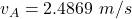The  gauge pressure is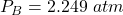Explanation:

The diagram for this question is shown on the first uploaded image

From the question we are told that

The gauge pressure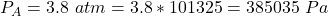The speed of flow is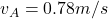The diameter of the pipe is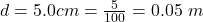The diameter at the top floor is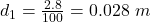The the height from the ground is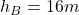So we are going to make some assumption

We would assume that the position on the street is A

and the position on the top floor is B

So from continuity equation the velocity of the flow in the street is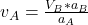Where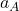is the area of the pipe at the base

So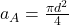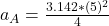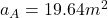and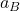i the area of the pipe at the top floor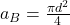substituting values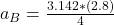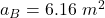So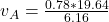Applying Bernoulli’s equation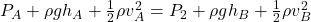Since the pipe started from the floorHere the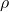is the density of water with value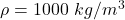Substituting values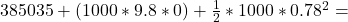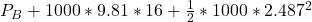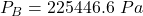Converting back to atm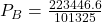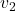= 2.49 m/s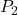= 2.19 atm

Explanation:

By using continuity equation:= ($$v_1$$) /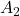= (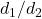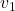= (5/2.8)² x 0.78 = 2.49 m/s

By using Bernoulli’s Equation: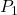+ ρg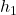+ ½ρ ()²=+ ρg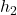+ ½ρ()²

[² –²] /2  = ()/ ρ + g()

(0.78²- 2.49²)/2 = ()/ 1000 + (9.8 x 16)

-5.6= ()/ 1000 + (156.8)

() = – 162400 Pa=-162400,= 3.8atm = 385035 Pa= 385035-162400 = 222635 Pa ( gauge  pressure)= 222635 Pa=> 2.19 atm